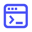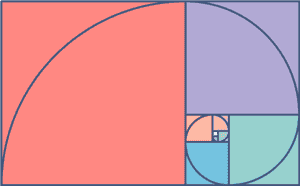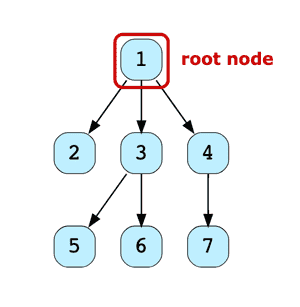# The insider's guide to recursion interview questions

Apr 16, 2019 - 6 min readEducativeTime and time again, you hear people say “Recursion is too hard”, or “Why do I need to learn recursion if it can be solved with iteration?” A simple Google search will find a vast number of questions asking why recursion is so difficult to understand.

So why learn recursion? Well, being able to think recursively is very important in programming for a couple of reasons:

• Often solving a problem with recursion is cleaner and easier to implement than if you were to do it iteratively. A good example of where recursion is useful is in QuickSort algorithms.

• It can be used to break down problems into smaller components — a recursive pattern known as Divide and Conquer. This is particularly useful for techniques such as MergeSort, binary search, and depth-first search.

Recursion is a fundamental problem-solving style and every developer should have it in their toolbox. You’d be surprised at how often recursion comes up in coding interviews, particularly at bigger companies like Google, Microsoft, and Facebook.

So if you’re heading into an interview and feel queasy about recursion, then it may be time to brush up on it, and we’ll help you.

Here’s what we’ll cover today:

## Process overview: Solving Fibonacci problem

One of the very first programs that gets you thinking about recursion is the Fibonacci Sequence. Let’s take a brief moment to look at an example in C++.

{
// base case
if (n<=1)
{
return n;
}
// recursive case
else
{
return (fibonacci(n-1) + fibonacci(n-2))
}
}

Here, we have the recursive function which contains two parts; the base case and the recursive case.

The base case of the function is being defined in line 5 where the function will terminate and return n when the if condition of n<=1 is met. In other terms, the base case is what terminates your program.

The recursive case comes next, or the function that we want to repeat until we have reached the base case. So if the base case condition does not evaluate to true, the function then enters the else block (line 8-11), where it then recursively calls itself with the input arguments fibonacci(n-1)+ fibonacci(n-2), as seen in line 10.

Now, your interviewer will tell you something like, "return the nth element in a Fibonacci series.”

Your first solution would likely be the brute-force while loop approach. This approach solves the problem but is not optimized.

So the interviewer asks, “how would you optimize this algorithm?” One way to do it would be through recursion seeing as you only have two variables (fibonacci n-1) and (fibonacci n-2).

They may ask a follow-up question such as, “how would you further optimize your recursive function?” One way to do this would be through memoization — a technique used to speed up computer programs by storing the results of expensive function calls and returning the cached result when the same inputs occur again.

What is great about this example and the Fibonacci sequence is that it is a transition into some very useful techniques like memoization and dynamic programming.## Tips for approaching recursion problems

### Always think about the base case

If you’re in a technical interview and a recursion question comes up, it is always best to begin with the end in mind or the base case. There are two parts to a recursive function;

• The first is a base case, where the call to the function stops i.e., it does not make any subsequent recursive calls.
• The second part to a recursive function is the recursive case, where the function calls itself again and again until it reaches the base case.

It’s important to remember that your base case ensures that the program will exit. Otherwise, your program will run infinitely.

### How to recognize a recursion question

Remember back to the days of probabilities and word problems? How they taught you whenever you heard the word “and” you would multiply the probabilities and “or” meant you added them. This concept has some similarities to programming and in particular recursion.

A really good indication of when you should use recursion is when you hear concepts such as “tree”, “graph”, “linked list”, or “nested”. These are almost dead giveaways that you’ll need to perform some recursive function.

### Questions to consider as you work

If you’re being asked a technical question in an interview that deals with recursion it’s important to be thinking about a few things:

• What is the base case?
• What is the simplest recursive case I can solve for? Then expand on it from there.
• How does your approach to solving the problem affect the stack? Then think of ways to improve on it.

## Concepts to studyData Structures

• Heaps: Tree-based data structure in which the tree is a complete binary tree.
• Trees: Composed of a collection of nodes where the first node in a tree is called the root and the last node is called a leaf.
• Graph: Non-linear data structure consisting of nodes and edges and is used to represent networks.
• Linked lists: Linear collection of data elements, whose order is not given by their physical placement in memory.

Algorithms

• Graph Traversals: Depth first search and Breadth First Search
• Quicksort: Highly efficient sorting algorithm and is based on partitioning an array of data into smaller arrays.
• Mergesort: Breaks down a list into several sublists until each sublist consists of a single element and merging those sublists in a manner that results into a sorted list.
• Binary search: Finds the position of a target value within a sorted array recursively working on a smaller and smaller array until you either find the value or the array size becomes 0.

Patterns

• Divide and conquer — a pattern that breaks the problem into smaller subproblems which are then solved for recursively and combined (great for tree sorting).

Techniques

• Memoization: Used to speed up computer programs by storing the results of expensive function calls and returning the cached result when the same inputs occur again.
• Backtracking: Considers searching every possible combination in order to solve an optimization problem.

## Recursion practice problems

In order to feel comfortable in a technical interview, you must practice these concepts that are mentioned above. Here are a few sample problems you can work through and get you thinking about recursion:

Problem Statement:

Print a reverse linked list. Start off by keeping it simple. Try printing this linked list in reverse.Problem Statement:

Depth-first search in graphs. This will get you familiar with traversal algorithms. Try writing some recursive code for this graph## What to learn next

Recursion produce cleaner, more efficient code, and demonstrates that you can think about problems in different ways.

There are a few things you’ll want to prepare for recursion interview questions:

1. Understand the basics: Understand why recursion matters, and where it’s useful and where it isn’t.

2. Convert solutions Iterative to Recursive: Practice converting unoptimized iterative solutions to optimized recursive solutions.

3. Practice: Practice makes perfect. Try writing out iterative and recursive solutions on a whiteboard and talk through how you did it.

To help you with these steps, Educative has put together a Recursion for Coding Interviews collection, with courses for all the top languages. Each course covers advanced recursion concepts and gives you hands-on practice with top recursion interview questions.

Happy learning!